# Selina Concise Physics Class 10 ICSE Solutions Radioactivity

## Selina Concise Physics Class 10 ICSE Solutions Radioactivity

APlusTopper.com provides step by step solutions for Selina Concise ICSE Solutions for Class 10 Physics Chapter 12 Radioactivity. You can download the Selina Concise Physics ICSE Solutions for Class 10 with Free PDF download option. Selina Publishers Concise Physics for Class 10 ICSE Solutions all questions are solved and explained by expert teachers as per ICSE board guidelines.

Selina ICSE Solutions for Class 10 Physics Chapter 12 Radioactivity

Exercise 12(A)

Solution 1.

Three constituent of an atom are:

1. Electrons: mass is 9.1 x 10-31 kg, charge is -1.6 x 10-19C
2. Neutron: mass is 1.6749 x 10-27 kg, charge is zero.
3. Protons: mass is 1.6726 x 10-27 kg, charge is +1.6 x 10-19 C

Solution 2.

Atomic number – the number of protons in the nucleus is called atomic number.
Mass number – the total number of nucleons in the nucleus is called mass number.

Solution 3.

• The nucleus at the centre of atom, whose size is of the order of 10-15 m to 10-14 m.
• The size of a nucleus is 10-5 to 10-4 times the size of an atom. It consists of protons and neutrons.
• If Z is the atomic number and A is the mass number of an atom, then the atom contains Z number of electrons; Z number of protons and A – Z number of neutrons.
• The atom is specified by the symbol ZXwhere X is the chemical symbol for the element.

Solution 4.

Atomic number Z = 11
Mass number A = 23
Number of neutrons A – Z = 12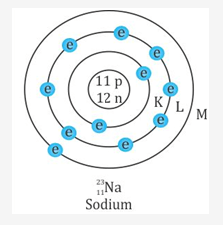Solution 5.

Isotopes: the atoms of the same element which have the same atomic number Z but differ in their mass number A are called isotopes.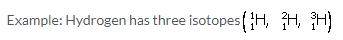Solution 6.

Isobars: the atoms of different elements which have the same mass number A, but differ in their atomic number Z are called isobars.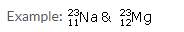Solution 7.Solution 8.

Radioactivity: Radioactivity is a nuclear phenomenon. It is the process of spontaneous emission of α or β and γ radiations from the nuclei of atoms during their decay.

Solution 9.

There will be no change in the nature of radioactivity. This is because radioactivity is a nuclear phenomenon.

Solution 10.

(a) Three types of radiations: Alpha, beta and gamma.

Solution 11.

(a) Gamma radiations have zero mass.
(b) Gamma radiations have the lowest ionizing power.
(c) Alpha particles have lowest penetrating power.
(d) Alpha particle has positive charge equal to 3.2 x 10-19C and rest mass equal to 4 times the mass of proton i.e. 6.68 x 10-27 kg.
(e) The gas is Helium.(f) These radiations come from nucleus of the atom.

Solution 12.

• Radiations labeled A, B and C are α, β and γ respectively.
• Radiation labeled A is gamma radiation because they have no charge and hence under action of magnetic field they go undeflected.
• Radiation B is alpha radiation because its mass is large and it would be deflected less in comparison to beta radiation. The direction of deflection is given by Fleming’s left hand rule. Also directions of deflection of alpha and beta radiations are opposite as they have opposite charge.

Solution 13.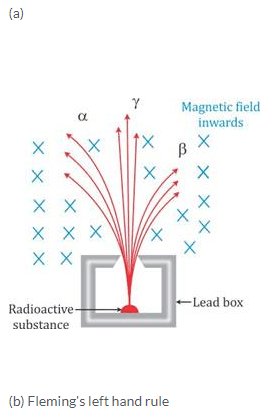Solution 14.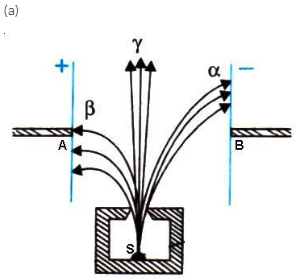(b) The radioactive substances are kept in thick lead containers with a very narrow opening, so as to stop radiations coming out from other directions because they may cause biological damage.

Solution 15.

This is because alpha and beta particles are charged particles, but gamma rays are neutral particles.

Solution 16.

No, it is not possible to deflect gamma radiation in a way similar to alpha and beta particles, using the electric or magnetic field because they are neutral and hence do not deflected under the action of electric or magnetic field.

Solution 17.

 Property α-particle β-particle γ-particle Nature Stream of positively charged particles, i.e. helium nuclei. Stream of negatively charged particles, i.e. energetic electrons. Highly energetic electromagnetic radiation. Charge Positive charge (Two times that of a proton) = + 3.2 x 10-19 C (or +2e) Negative charge = – 1.6 x 10-19 C (or -e) No charge Mass Four times the mass of proton i.e., 6.68 x 10-27 kg Equal to the mass of electron, i.e. 9.1 x 10-31 kg No mass (Rest mass is zero) Effect of electric field Less deflected More deflected than alpha particles but in direction opposite to those of α particles Unaffected

Solution 18.

Ionizing power of alpha radiation is maximum i.e., 10000 times of gamma radiation while beta particles have lesser ionizing power i.e., 100 times of gamma radiation and gamma radiation have least ionizing power.
Penetration power is least for alpha particle and maximum for gamma radiation.

Solution 19.

• Speed of α radiation is nearly 107 m/s.
• Speed of β radiation is about 90% of the speed of light or 2.7 x 108 m/s.
• Speed of γ radiation is 3 x 108 m/s in vacuum.

Solution 20.

• Alpha radiations are composed two protons and two neutrons.
• Beta particles are fast moving electrons.
• Gamma radiations are photons or electromagnetic waves like X rays.
• Alpha radiations have the least penetrating power.

Solution 21.

• Gamma radiation are produced when a nucleus is in a state of excitation (i.e., it has an excess of energy). This extra energy is released in the form of gamma radiation.
• Gamma radiations like light are not deflected by the electric and magnetic field.
• Gamma radiations have the same speed as that of light.

Solution 22.

It will become singly ionized helium He+.

Solution 23.

Any physical changes (such as change in pressure and temperature) or chemical changes (such as excessive heating, freezing, action of strong electric and magnetic fields, chemical treatment, oxidation etc.) do not alter the rate of decay of the radioactive substance. This clearly shows that the phenomenon of radioactivity cannot be due to the orbital electrons which could easily be affected by such changes. The radioactivity should therefore be the property of the nucleus. Thus radioactivity is a nuclear phenomenon.

Solution 24.

On emitting a β particle, the number of nucleons in the nucleus (i.e. protons and neutrons) remains same, but the number of neutrons is decreased by one and the number of protons is increased by one.

If a radioactive nucleus P with mass number A and atomic number Z emits a beta particle to form a daughter nucleus Q with mass number A and atomic number Z+1, then the change can be represented as follows:(a) Atomic number ‘Z’ is not conserved. It is increased by 1.
(b) Mass number A is conserved.

Solution 25.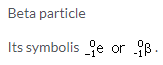Solution 26.

(a) Atomic number decreases by 2.
(b) Atomic number increases by 1.
(c) Atomic number does not change.

Solution 27.

(a) After emitting an alpha particle the daughter element occupies two places to the left of the parent element in the periodic table.
Reason: If a parent nucleus X becomes a new daughter nucleus Y as a result of α-decay, then the α-decay can be represented as:Thus, the resulting nucleus has an atomic number equal to (Z-2). Hence, it shifts two places to the left of the parent element in the periodic table.

(b) After emitting a -particle, the daughter element occupies one place to the right of the parent element in the periodic table.
Reason: If a parent nucleus X becomes a new daughter nucleus Y as a result of β-decay, then the β-decay can be represented as: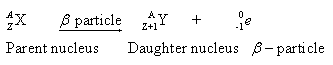Thus, the resulting nucleus has an atomic number equal to (Z+1). Hence, it shifts one place to the right of the parent element in the periodic table.

(c) After emitting -radiation, the element occupies the same position in the periodic table.
Reason: If a parent nucleus X becomes a new daughter nucleus Y as a result of γ-decay, then the γ-decay can be represented as: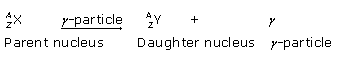Thus, the resulting nucleus has atomic number equal to Z. Hence, it occupies the same position as the parent element in the periodic table.

Solution 28.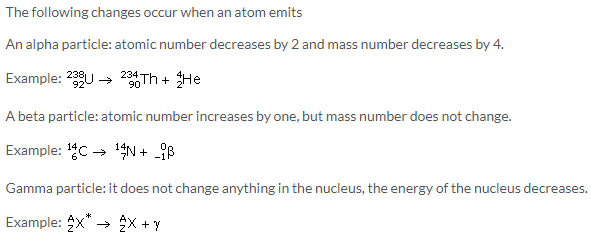Solution 29.

(a) The composition of B – 82 protons and 126 neutrons.
(b) The composition of C – 83 protons and 125 neutrons.
(c) The mass number of nucleus A = no. of protons +no. of neurons = 84+128=212.
(d) Their will be no change in the composition of nucleus C.

Solution 30.

(a) The alpha particle was emitted.
(b) This is because the atomic number has decreased by 2 and mass number has decreased by 4.Solution 31.

(a) This is allowed.
(b) This is not allowed because mass number is not conserved.

Solution 32.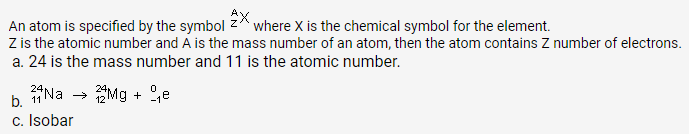Solution 33.Solution 34.

The atomic number of P decreases by 2 and mass no. decreases by 4 due to the emission of one alpha particle and then increases by 1 due to the emission of each beta particle, so the atomic number of Q formed after the emission of one alpha and two beta particles is same as that of P. Hence P and Q are the isotopes.

Solution 36.

(a) The mass number (A) of an element is not changed when it emits beta and gamma radiations.
(b) The atomic number of a radioactive element is not changed when it emits gamma radiations.
(c) During the emission of a beta particle, the mass number remains same.

Solution 37.Solution 38.

Radio isotopes: The isotopes of some elements with atomic number Z
Example: carbon (Z=6, A=14).
Radio isotopes are used in medical and scientific and industrial fields. Radio isotopes such as 92U232 are used as fuel for atomic energy reactors.

Solution 39.

Because they cannot penetrate the human skin.

Solution 40.

Gamma radiations have very high penetration power and can easily pass through the human body. Therefore they are used as radioactive tracers in medical science.

Solution 41.

When the number of neutrons exceeds much than the number of protons in a nuclei, it become unstable or radioactive.

Solution 42.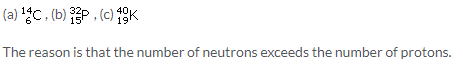Solution 43.

Many diseases such as leukemia, cancer, etc., are cured by radiation therapy. Radiations from cobalt -60 are used to treat cancer by killing the cells in the malignant tumor of the patient.

Solution 44.

a  <  β  <  γ

An α-particle rapidly loses its energy as it moves through a medium and therefore its penetrating power is quite small. It can penetrate only through 3 – 8 cm in air. It can easily be stopped by a thin card sheet or a thick paper.

The penetrating power of β-particles is more than that of the α-particles. They can pass through nearly 5 m in air, through thin card sheet, and even through thin aluminium foil, but a 5 mm thick aluminium sheet can stop them.

Whereas, the penetrating power of γ-rays is high. It is about 104 times that of α-particles and 102 times that of β-particles. They can pass through 500 m in air or through 30 cm thick sheet of iron. Thick sheet of lead is required to stop them.

Solution 45.

Two main sources of nuclear radiations are:

1. Radioactive fallout from nuclear plants and other sources.
2. Disposal of nuclear waste.

These radiations are harmful because when these radiations falls on the human body, they kill the human living tissues and cause radiation burns.

Solution 46.

The following safety measures must be taken in a nuclear power plant:

1. The nuclear reactor must be shielded with lead and steel walls so as to stop radiations from escaping out to the environment during its normal operation.
2. The nuclear reactor must be housed in an airtightbuilding of strong concrete structure which can withstand earthquakes, fires and explosion.
3. There must be back up cooling system for the reactor core, so that in case of failure of one system, the other cooling system could take its place and the core is saved from overheating and melting.

Solution 47.

The radioactive material after its use is known as nuclear waste.
It must be buried in the specially constructed deep underground stores made quite far from the populated area.

Solution 48.

Three safety precautions that we would take while handling the radioactive substances are:

3. Keep the radioactive substances in thick lead containers with a very narrow opening, so as to stop radiations coming out from other directions.

Solution 49.

Radioactive substance should not be touched by hands because these radiations are harmful; when radiation falls on the human body, they kill the human living tissues and cause radiation burns.

Solution 50.

Background radiation: These are the radioactive radiations to which we all are exposed even in the absence of an actual visible radioactive source.
There are two sources of background radiation:

1. Internal source: potassium, carbon and radium are present inside our body.

It is not possible for us to keep ourselves away from the background radiations.

Solution 1 (MCQ).

α or β
Hint: In a single radioactive decay, α and β particles are never emitted simultaneously. There will be either an α-emission or a α β-emission, which may be accompanied by γ emission.

Solution 2 (MCQ).

The nucleus of the atom.
Hint: Radioactivity is a nuclear phenomenon. Hence, electrons come out from the nucleus. Electron is created as a result of decay of one neutron into a proton inside the nucleus and it is not possible for the electron to stay inside the nucleus; thus, it is spontaneously emitted.

Solution 3 (MCQ).

(a) α – particles

An α – particle rapidly loses its energy as it moves through a medium and therefore its penetrating power is quite small. It can penetrate only through 3 – 8 cm in air. It can easily be stopped by a thin card sheet or a thick paper.

Solution 4 (MCQ).

(b) β-particles

β-particles are negatively charged, so they get deflected by the electric and magnetic fields. The deflection of β-particle is more than that of a-particle since a β-particle is lighter than the α-particle. Whereas, gamma radiations are not deflected by the electric and magnetic fields since they are not charged particles.

Exercise 12(B)

Solution 1.
Energy released by combining of nuclei of an atom or by decay of an unstable radioactive nucleus during a nuclear reaction i.e., during fusion or fission is known as nuclear energy.

Solution 1 (MCQ).
(d) neutron
A neutron is used in nuclear fission for bombardment.

Solution 1 (Num).
1 a.m.u. = 1.66 × 10-27 kg
→ 0.2 a.m.u. = 0.2 × 1.66 × 10-27 kg
Δm = 0.332 Δ 10-27 kg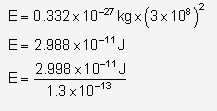Solution 2.
Einstein’s mass-energy equivalence relation : E = Δmc2
Where E is the energy released due to the loss in the mass Δm and c is the speed of light.

Solution 2 (MCQ).
(d) 10K
To make the fusion possible, a high temperature of approximately 107 K and high pressure is required.

Solution 2 (Num).
Given that Δm = 0.0265 a.m.u.
1 a.m.u. liberates 931.5 MeV of energy. Thus, energy liberated equivalent to 0.0265 a.m.u. is
= 0.0265 a.m.u. × 931.5 MeV
= 24.7 meV

Solution 3.
(a) The mass of atomic particles is expressed in terms of atomic mass unit (a.m.u.). 1 a.m.u. of mass is equivalent to 931 MeV of energy.
(b) Mass of proton = 1.00727 a.m.u.
Mass of neutron = 1.00865 a.m.u.
Mass of electron = 0.00055 a.m.u.

Solution 4.
Nuclear fission is the process in which a heavy nucleus is splits into two light nuclei nearly of the same size by bombarding it with slow neutrons.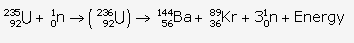Solution 5.
(a) $$_{ 92 }^{ 235 }{ U }$$ and $$_{ 92 }^{ 235 }{ U }$$
(b) Experimentally it is found that isotope of $$_{ 92 }^{ 235 }{ U }$$ is more easily fissionable because the fission of
is $$_{ 92 }^{ 235 }{ U }$$ possible by sloe neutron unlike $$_{ 92 }^{ 238 }{ U }$$ where fission is possible only by the fast neutrons.
(c) Slow and fast both.

Solution 6.
Nearly 190 MeV of energy is released due to fission of one nucleus of $$_{ 92 }^{ 235 }{ U }$$ The cause of emission of this energy is the loss in mass i.e., the sum of masses of product nuclei is less than the sum of mass of the parent nucleus and neutron.

Solution 7.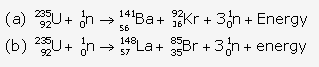Solution 8.
A chain reaction is a series of nuclear fissions whereby the neutrons produced in each fission cause additional fissions, releasing enormous amount of energy.
It is controlled by absorbing some of the neutrons emitted in the fission process by means of moderators like graphite, heavy water, etc. then the energy obtained in fission can be utilized for the constructive purposes

Solution 9.
(i) It is used in a nuclear bomb.
(ii) It is used in a nuclear reactor where the rate of release of energy is slow and controlled which is used to generate electric power.

Solution 10.

 Radioactive decay Nuclear Fission It is a self process. It does not occur by itself. Neutrons are bombarded on a heavy nucleus. The nucleus emits either the a or b particles with the emission of energy in form of g rays which is not very large. A tremendous amount of energy is released when a heavy nucleus is bombarded with neutrons and the nucleus splits in two nearly equal fragments. The rate of radioactive decay cannot be controlled. The rate of nuclear fission can be controlled.

Solution 11.
Nuclear fission is the process in which a heavy nucleus is splits into two light nuclei nearly of the same size by bombarding it with slow neutrons.
When uranium with Z = 92 is bombarded with neutron, it splits into two fragments namely barium (Z = 56) and krypton (Z = 36) and a large amount of energy is released which appears due to decrease in the mass.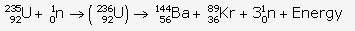Nuclear fusion is also known as thermo-nuclear reaction. This is because nuclear fusion takes place at very high temperature.

Solution 12.
When two nuclei approach each other, due to their positive charge, the electrostatic force of repulsion between them becomes too strong that they do not fuse. Thus, nuclear fusion is not possible at ordinary temperature and ordinary pressure.
Hence to make the fusion possible, a high temperature of approximately 107 K and high pressure is required. At such a high temperature, due to thermal agitations both nuclei acquire sufficient kinetic energy so as to overcome the force of repulsion between them when they approach each other, and so they get fused.

Solution 13.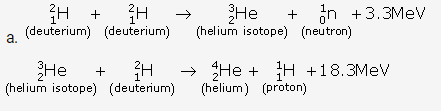(b) In all three deuterium nuclei fuse to form a helium nucleus with a release of 21·6 MeV energy.
(c) When two deuterium nuclei ($$_{ 1 }^{ 2 }{ H }$$) fuse, nucleus of helium isotope ($$_{ 3 }^{ 2 }{ He }$$ ) is formed and 3·3 MeVenergy is released. This helium isotope again gets fused with one deuterium nucleus to form a helium nucleus ($$_{ 4 }^{ 2 }{ He }$$
) and 18·3 MeV of energy is released in this process.

Solution 14.
(a)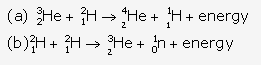Solution 15.
(a) Nuclear fusion
(b) Nuclear fission

Solution 16.
Both fission and fusion create release of neutrons and large amount of energy.
Nuclear fission: A heavy nucleus splits in two nearly equal light fragments when bombarded with neutrons. It is possible at very ordinary temperature and pressureNuclear fusion: Two light nuclei combine to form a heavy nucleus at very high temperature and high pressure. Possible only at a very high temperature (≈107 K) and a very high pressure.

Solution 17.Solution 18.
The source of energy in the Sun and stars is the nucleus fusion of light nuclei such as hydrogen present in them in their inner part. This takes place at a very high temperature and high pressure due to which helium nucleus is formed with the release of high amount of energy.

Solution 19.
(a) Nuclear fission
(b) Nuclear fusion

More Resources for Selina Concise Class 10 ICSE Solutions